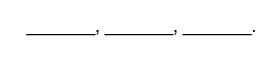# Algebra 2 - Permutations

## Introduction - Fundamental Counting Principle and Permutation

• The fundamental counting principle states that if one thing can occur in $m$ ways, a second occurs in $n$ ways, and a third thing can occur in $r$ ways, then the sequence of things can occur in $m·n·r$ ways.
• Factorial Notation defines that the product of the positive integers from  inclusive occurs typically in the field of mathematics and is denoted by the notation $\mathbit{n}\mathbf{!}$, which is termed orally as
• It is a given fact that $0!$ = 1.
• $n!=n\left(n-1\right)\left(n-2\right)\left(n-3\right)...\left(3\right)\left(2\right)\left(1\right)$
• A Permutation is an arrangement of $n$ objects in a given order. An arrangement of any $r\le n$ of these objects in a given order is a permutation of $n$ taken $r$ at a time.
• Generally, the formula of Permutation is given by ${}_{n}P_{r}=\frac{n!}{\left(n-r\right)!}$ or .

Some Solved Examples involving the Fundamental Counting Principle:

Example 1. How many three-digit numbers can be formed using the digits  if no digit is repeated?

Solution: We can count the total possible permutations of three-digit numbers by using the blanks below that describe the position of the unit digit, the tens digit, and the hundreds digit.The hundredth digit can be done in 3 ways since 0 can't be the first digit. The tenth digit can be done in 3 ways. The units digit can be done in 2 ways.

Using the fundamental counting principle, we have:

Example 2. How many four-digit even numbers greater than 3,000 can be formed using only the digits 1, 2, 3, and 4 if repetition is allowed?

Solution:

Use the concept of the Fundamental Counting Principle.

For four-digit numbers, we have: $2·4·4·2=64$

For three-digit numbers, we have: $4·4·2=32$

For two-digit numbers, we have: $4·2=8$

One-digit numbers can have two numbers only.

Thus, the total number of four-digit even numbers formed greater than 3,000 is computed as: $64+32+8+2=106$.

## Solved Examples

Consider the letters This means all their possible arrangements are . This implies permutation 3 taken 3, which is 6.

Example 1. Evaluate ${}_{7}P_{4}$?

Using the formula ${}_{n}P_{r}=\frac{n!}{\left(n-r\right)!}$, we have

${}_{7}P_{4}=\frac{7!}{\left(7-4\right)!}=\frac{7·6·5·4·3·2·1}{3·2·1}=840$

Example 2. Find the number of permutations of 7 letters, taken three at a time.

Using the Fundamental Counting Principle, we have: ${n}_{1}·{n}_{2}·{n}_{3}=7·6·5=210$

Using Permutation, we have: $P\left(7,3\right)=\frac{7!}{\left(7-3\right)!}=210$

Example 3. In how many ways can the letters in the word ENGINEERING be arranged?

Letters E and N are repeated three times, and letters I and N are repeated twice.

Example 4. How many ways can one select three cards in succession from a deck of cards with replacements? Without replacement?

With Replacement:

Without Replacement:

Example 5. Suppose nine mathematics and six physics books will be arranged on a shelf. How many ways can such books be arranged if the books of the same subject shall be placed side by side?

$P\left(9,9\right)·P\left(6,6\right)·2!=522,547,200$

Example 6. How many ways can 7sevenmen be seated at a circular table such that two men must always sit beside each other?

## Cheat Sheet

• The formula $P={n}^{r}$ is used when order is important to compute permutations and repetition is allowed.
• The formula ${}_{n}P_{r}=\frac{n!}{\left(n-r\right)!}$ is used when order matters and repetition is not allowed.

## Blunder Areas

• The number of permutations is larger than the number of combinations.
• The concept of permutations stresses that order matters, while the concept of combinations uses no order of arrangement.
• $0!\ne 0$. It is a basic idea that $0!=1$.
• In the formula $r\le n$.
• In solving problems involving permutations and combinations, always be careful in analyzing the type of situation involved, whether it involves an order of arrangement or not.